Test: EDC & Analog

# Test: EDC & Analog - Electronics and Communication Engineering (ECE)

Test Description

## 25 Questions MCQ Test GATE ECE (Electronics) 2024 Mock Test Series - Test: EDC & Analog

Test: EDC & Analog for Electronics and Communication Engineering (ECE) 2023 is part of GATE ECE (Electronics) 2024 Mock Test Series preparation. The Test: EDC & Analog questions and answers have been prepared according to the Electronics and Communication Engineering (ECE) exam syllabus.The Test: EDC & Analog MCQs are made for Electronics and Communication Engineering (ECE) 2023 Exam. Find important definitions, questions, notes, meanings, examples, exercises, MCQs and online tests for Test: EDC & Analog below.
Solutions of Test: EDC & Analog questions in English are available as part of our GATE ECE (Electronics) 2024 Mock Test Series for Electronics and Communication Engineering (ECE) & Test: EDC & Analog solutions in Hindi for GATE ECE (Electronics) 2024 Mock Test Series course. Download more important topics, notes, lectures and mock test series for Electronics and Communication Engineering (ECE) Exam by signing up for free. Attempt Test: EDC & Analog | 25 questions in 75 minutes | Mock test for Electronics and Communication Engineering (ECE) preparation | Free important questions MCQ to study GATE ECE (Electronics) 2024 Mock Test Series for Electronics and Communication Engineering (ECE) Exam | Download free PDF with solutions
 1 Crore+ students have signed up on EduRev. Have you?
Test: EDC & Analog - Question 1

### The figure Shows V-I charactertics of a solar cell illuminated uniformly with solar light of power 100mW/cm2. The solar cell has an area of 3cm2 and a fill factor of 0.7. The maximum efficiency of cell is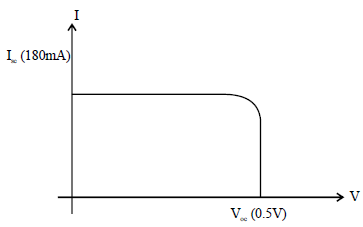Test: EDC & Analog - Question 2

### In the circuit shown below, assume that the diodes D1 and D2 are ideal. The average value of voltage Vab (in volts) across the terminals a and b is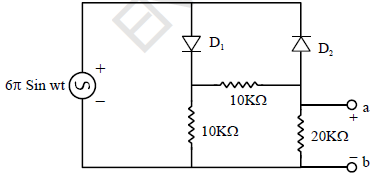Test: EDC & Analog - Question 3

### Consider an n-type semiconductor in which the dopant concentration ND is 1014/cm3. If the intrinsic concentration of semiconductor 1010/cm3 then the concentration of electrons (n) and holes (P) at equillibrium are

Test: EDC & Analog - Question 4

Consider the following statements:

1. Fermi level in a p-type semiconductor lies close to the top of the valence band.

2. The forbidden energy in Germanium at 0 K is exactly 0.78 eV.

3. When a p-n junction is reverse biased, then electrons and holes move away from the junction.

Which of these statements are correct?

Detailed Solution for Test: EDC & Analog - Question 4

Fermi level:

The Fermi level is the energy separating occupied states of the valence band from empty states of the conduction band at the temperature T=0 K.

Fermi energy level in a p-type semiconductor:

For a p-type semiconductor, there are more holes in the valence band than there are electrons in the conduction band.

This implies that the probability of ﬁnding an electron near the conduction band edge is smaller than the probability of ﬁnding a hole at the valence band edge.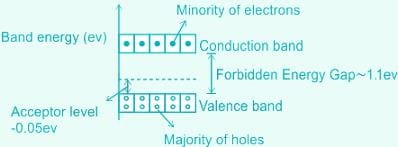Therefore, the Fermi level is closer to the valence band in a p-type semiconductor.

Forbidden energy gap:

It is nothing but the difference between the conduction energy band and valence energy band For Germanium, the forbidden energy gap is 0.78 eV.

P-N diode:

A p-n diode is a two-terminal semiconductor device that

1. Allows the current in only one direction (forward biasing)
2. Blocks the current in the opposite direction (reverse biased).

Reverse bias:

When a p-n junction is reversed biased

1. The negative terminal of the battery attracts the free holes in the p-type towards itself
2. The positive terminal attracts the free electrons in the n-type towards itself.
3. Hence, the electrons and the holes move away from the junction
4. Due to this, the depletion width as well as the potential barrier are increased.
Test: EDC & Analog - Question 5

The junction capacitance of an abrupt pn junction is 10 pf at a reverse bias voltage of 3 volt. Determine the value of capacitance when a reverse bias voltage of 15 volt is applied, if the built in potential of junction is 1 volt.

Test: EDC & Analog - Question 6

In the circuit shown, diodes D1, D2 and D3 are ideal and the inputs E1, E2 and E3 are 0 V for logic ‘0’ and 5V for logic ‘1’. What logic gate does the circuit represent?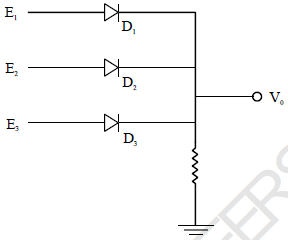Test: EDC & Analog - Question 7

In the given circuit, the moving-coil meter gives full-scale deflection reading when the average current
flowing through it is 1mA. The moving coil meter has internal resistance of 50?. Find the value of R that results meter a full scale deflection.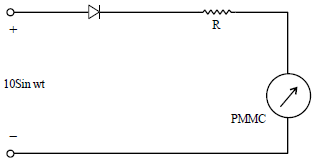Test: EDC & Analog - Question 8

Assume both the diodes and both the capacitors are ideal. The value of V0 in steady state is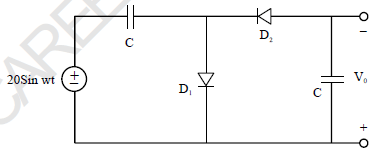Test: EDC & Analog - Question 9

Assume the diode is ideal, determine the current ‘i’ in the given circuit.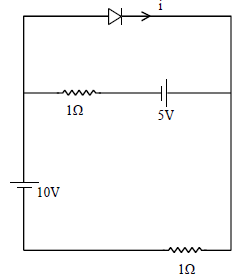Test: EDC & Analog - Question 10

Consider the following statements.

1. The PIV in a HWR with capacitive filter becomes 2 Vm

2. Ripple voltage is decreases as we increases the time period of input ac signal.

3. Conduction time of diode is decreases as we increase the value of capacitor.

Which of the following is/are correct?

Test: EDC & Analog - Question 11

In the given circuit both the transistors are perfectly matched the value fo I2 is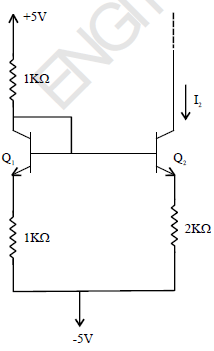Test: EDC & Analog - Question 12

The forward threshold voltage of each diode in the given circuit is 1 Volt and reverse breakdown voltage of each diode is 10 Volt. Determine ‘i’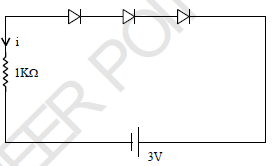Test: EDC & Analog - Question 13

In the given circuit the VCE (sat) for each transistor is 0. The inputs A, B and C are 0 for logic 0 and they
are 5V for logic 1. The logic gate realized by the circuit. Assume β = 100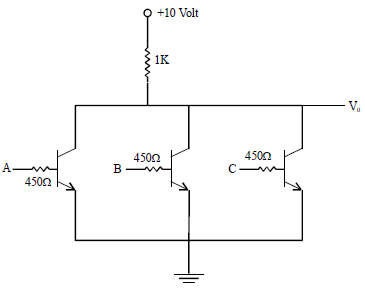Test: EDC & Analog - Question 14

Assume β of the transistor is 100, determine the value of V0 in the given circuit.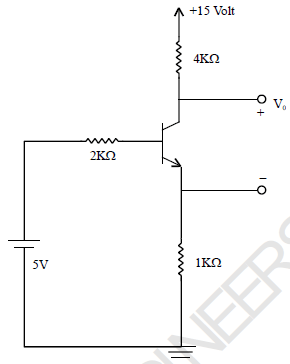Test: EDC & Analog - Question 15

In the ac circuit shown {The DC biasing circuit is omitted), the two BJTs are biased in active region and
have identical parameters with β >> 1. The open circuit small signal voltage gain is approximately.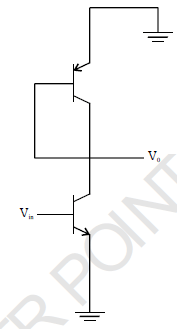Test: EDC & Analog - Question 16

In the circuit shown below, assume both the diodes and opamp are ideal. Determine the frequency of the oscillation of the signal obtained at V0.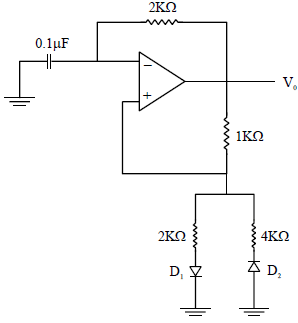Test: EDC & Analog - Question 17

In the given circuit, assume opamp is ideal. Determine the type and 3-dB frequency of the filter.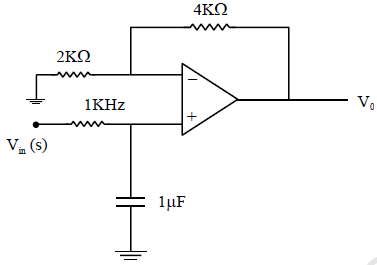Test: EDC & Analog - Question 18

For the CMOS circuit shown below the parameters for the MOSFET are given below:
For NMOS : Vth = 0.7V,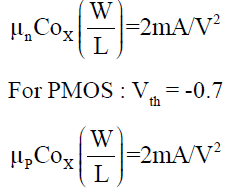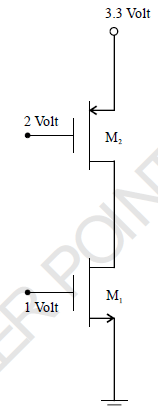Determine region of MOSFET M1 and M2.

Test: EDC & Analog - Question 19

For the MOSFET shown in the circuit below has Vth = 1 Volt,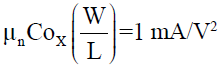. Determine the resistance offered by the MOSFET.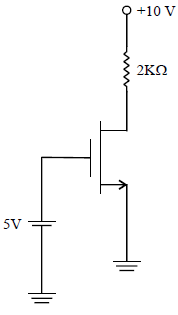Test: EDC & Analog - Question 20

Which of the following statement is not correct about a power amplifier?

Test: EDC & Analog - Question 21

Determine the duty cycle of the square wave generated at the output of terminal 3: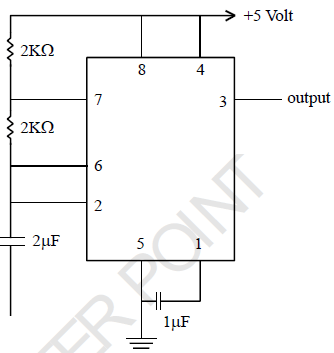Test: EDC & Analog - Question 22

A light of wavelength 4000A is incident on a semiconductor material having energy band gap is 1.4eV and electron hole pairs are generated. Determine the velocity of electron that is generated due to this illumination.

Test: EDC & Analog - Question 23

In the given circuit the opamp is ideal.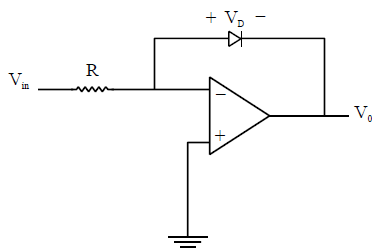This circuit is popularly known as,

Test: EDC & Analog - Question 24

Adding an emitter resistor to a common-emitter amplifier causes:

Test: EDC & Analog - Question 25

A non-inverting op-amp is shown below {assume ideal op-amp}. For
Vin = (2 + Sin 100t) Volt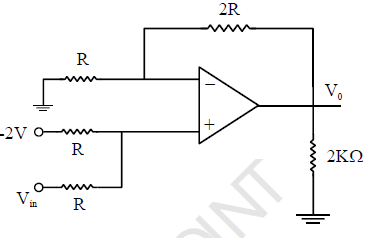The output voltage V0 for an input

## GATE ECE (Electronics) 2024 Mock Test Series

22 docs|263 tests
Information about Test: EDC & Analog Page
In this test you can find the Exam questions for Test: EDC & Analog solved & explained in the simplest way possible. Besides giving Questions and answers for Test: EDC & Analog, EduRev gives you an ample number of Online tests for practice

## GATE ECE (Electronics) 2024 Mock Test Series

22 docs|263 tests